# Non Verbal Reasoning - Cubes and Dice

### Exercise :: Cubes and Dice - Section 1

The sheet of paper shown in the figure (X) given on the left hand side, in each problem, is folded to form a box. Choose from amongst the alternatives (1), (2), (3) and (4), the boxes that are similar to the box that will be formed.

11.

Choose the box that is similar to the box formed from the given sheet of paper (X).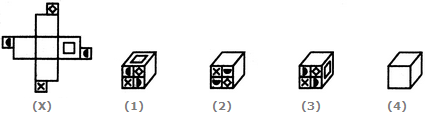A. 1, 2 and 3 only B. 2 and 3 only C. 1, 3 and 4 only D. 2, 3 and 4 only

Explanation:

The fig. (X) is similar to the Form VII. So, when a cube is formed by folding the sheet shown in fig. (X), then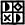is one of the faces of the cube and this face lies opposite to a blank face. Also, a face bearing a square lies opposite to another blank face. The remaining two blank faces lie opposite to each other. Clearly, in the cube shown in fig. (1), the face consisting of the four symbols is not the same as that formed (as shown above). Hence, the cube in fig. (1) cannot be formed.

12.

Choose the box that is similar to the box formed from the given sheet of paper (X).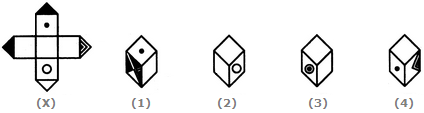A. 1 and 2 only B. 1, 2 and 3 only C. 1 and 3 only D. 1, 2, 3 and 4

Explanation:

The fig. (X) is similar to the Form VI. So, when a cube is formed by folding the sheet shown in fig. (X), then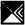is one of the faces of the cube and this face lies opposite to a blank face. Also, a face bearing a circle lies opposite to one bearing a dot. Clearly, this cube does not have faces as shown in the cubes in figures (3) and (4). Hence, only the cubes shown in figures (1) and (2) can be formed.

13.

Choose the box that is similar to the box formed from the given sheet of paper (X).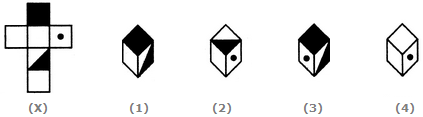A. 1 and 3 only B. 2, 3 and 4 only C. 2 only D. 3 and 4 only

Explanation:

The fig. (X) is similar to the Form I. So, when the sheet in fig. (X) is folded to form a cube, then the completely shaded face lies opposite to the half shaded face. Therefore, the cubes shown in figures (1) and (3) which have the completely shaded face adjacent to the half-shaded face cannot be formed. Since Fig 4 does't have at-least one shaded face, it cannot be formed. Hence, only the cubes in figure (2) can be formed.

14.

Choose the box that is similar to the box formed from the given sheet of paper (X).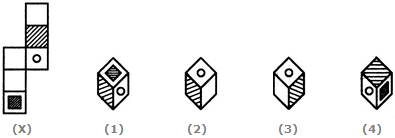A. 1 and 2 only B. 1, 2 and 4 only C. 1 and 4 only D. 1, 2 and 3 only

Explanation:

The fig. (X) is similar to the Form V. So, when the sheet shown in fig. (X) is folded to form a cube then the shaded face lies opposite to one of the blank faces, the face bearing a circle lies opposite to another blank face and the face bearing a shaded square lies opposite to the third blank face. Thus, each one of the cubes shown in figures (1), (2) and (4) can be formed. Also, though the cube shown in fig. (3) has faces that can appear adjacent to each other but the cube formed by folding the sheet in fig. (X) cannot be rotated to form fig. (3). Hence, the cube in fig.(3) cannot be formed.

15.

Choose the box that is similar to the box formed from the given sheet of paper (X).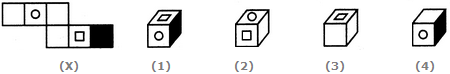A. 1 and 2 only B. 2, 3 and 4 only C. 4 only D. 3 and 4 only